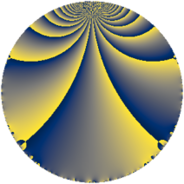# Properties

 Label 56.2.iLevel $56$ Weight $2$ Character orbit 56.i Rep. character $\chi_{56}(9,\cdot)$ Character field $\Q(\zeta_{3})$ Dimension $4$ Newform subspaces $2$ Sturm bound $16$ Trace bound $3$

# Related objects

## Defining parameters

 Level: $$N$$ $$=$$ $$56 = 2^{3} \cdot 7$$ Weight: $$k$$ $$=$$ $$2$$ Character orbit: $$[\chi]$$ $$=$$ 56.i (of order $$3$$ and degree $$2$$) Character conductor: $$\operatorname{cond}(\chi)$$ $$=$$ $$7$$ Character field: $$\Q(\zeta_{3})$$ Newform subspaces: $$2$$ Sturm bound: $$16$$ Trace bound: $$3$$ Distinguishing $$T_p$$: $$3$$

## Dimensions

The following table gives the dimensions of various subspaces of $$M_{2}(56, [\chi])$$.

Total New Old
Modular forms 24 4 20
Cusp forms 8 4 4
Eisenstein series 16 0 16

## Trace form

 $$4 q - 2 q^{3} + 2 q^{5} - 4 q^{9} + O(q^{10})$$ $$4 q - 2 q^{3} + 2 q^{5} - 4 q^{9} - 2 q^{11} - 8 q^{13} - 4 q^{15} + 2 q^{17} - 6 q^{19} - 2 q^{21} + 10 q^{23} + 8 q^{25} + 28 q^{27} - 8 q^{29} + 6 q^{31} + 6 q^{33} + 6 q^{35} + 2 q^{37} - 12 q^{39} - 24 q^{41} - 16 q^{43} + 4 q^{45} - 6 q^{47} + 4 q^{49} - 14 q^{51} + 10 q^{53} - 4 q^{55} + 28 q^{57} - 18 q^{59} + 2 q^{61} - 28 q^{63} - 4 q^{65} - 2 q^{67} - 4 q^{69} + 32 q^{71} - 14 q^{73} + 8 q^{75} + 14 q^{77} + 10 q^{79} - 10 q^{81} + 16 q^{83} + 4 q^{85} + 20 q^{87} + 2 q^{89} + 32 q^{91} - 2 q^{93} + 6 q^{95} + 8 q^{97} - 24 q^{99} + O(q^{100})$$

## Decomposition of $$S_{2}^{\mathrm{new}}(56, [\chi])$$ into newform subspaces

Label Dim $A$ Field CM Traces $q$-expansion
$a_{2}$ $a_{3}$ $a_{5}$ $a_{7}$
56.2.i.a $2$ $0.447$ $$\Q(\sqrt{-3})$$ None $$0$$ $$-3$$ $$1$$ $$4$$ $$q-3\zeta_{6}q^{3}+(1-\zeta_{6})q^{5}+(1+2\zeta_{6})q^{7}+\cdots$$
56.2.i.b $2$ $0.447$ $$\Q(\sqrt{-3})$$ None $$0$$ $$1$$ $$1$$ $$-4$$ $$q+\zeta_{6}q^{3}+(1-\zeta_{6})q^{5}+(-3+2\zeta_{6})q^{7}+\cdots$$

## Decomposition of $$S_{2}^{\mathrm{old}}(56, [\chi])$$ into lower level spaces

$$S_{2}^{\mathrm{old}}(56, [\chi]) \cong$$ $$S_{2}^{\mathrm{new}}(28, [\chi])$$$$^{\oplus 2}$$